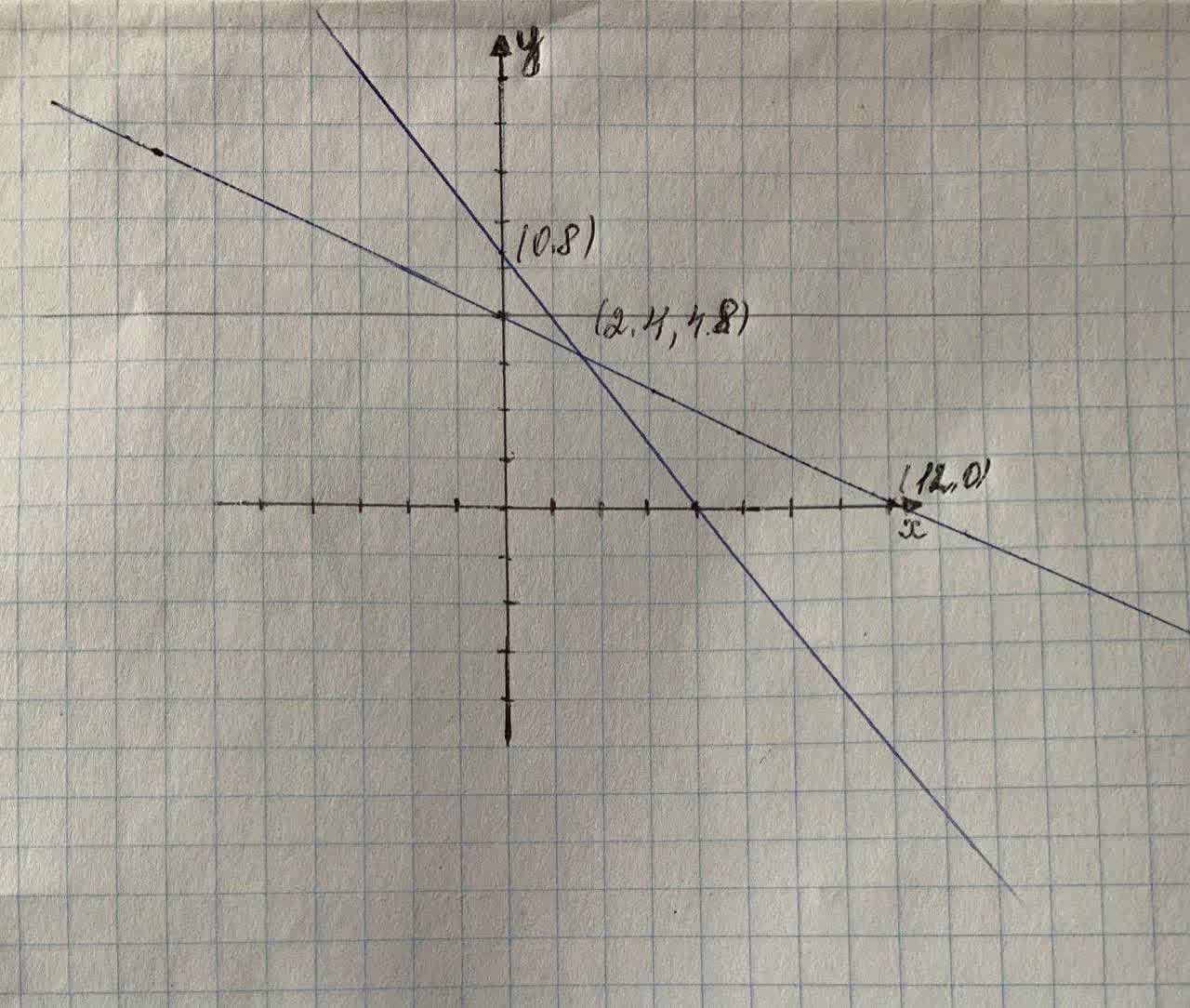# The Munchies Cereal Company makes a cereal from several ingredients. Ttbbfiladelfia6l 2021-11-23 Answered
The Munchies Cereal Company makes a cereal from several ingredients. Two of the ingredients, oats and rice, provide vitamins A and B. The company wants to know how many ounces of oats and rice it should include in each box of cereal to meet the minimum requirements of 48 milligrams of vitamin A and 12 milligrams of vitamin B while minimizing cost. An ounce of oats contributes 8 milligrams of vitamin A and 1 milligram of vitamin B, whereas an ounce of rice contributes 6 milligrams of A and 2 milligrams of B. An ounce of oats costs $0.05, and an ounce of rice costs$0.03.
a. Formulate a linear programming model for this problem.
b. Solve this model by using graphical analysis.
c. What would be the effect on the optimal solution if the cost of rice increased from $0.03 per ounce to$0.06 per ounce?

• Questions are typically answered in as fast as 30 minutes

### Plainmath recommends

• Get a detailed answer even on the hardest topics.
• Ask an expert for a step-by-step guidance to learn to do it yourself.navratitehf
Step 1
Only A and B part.
X= Ounces of Oats
Y= Ounces of Rice
$$\displaystyle{8}{X}+{6}{Y}\geq{48}$$ vitamin A
$$\displaystyle{1}{X}+{2}{Y}\geq{12}$$ vitamin B
$$\displaystyle{\cos{{t}}}={0.05}{X}+{0.03}{Y}$$Step 2
The critical values are on the graph
The costs at each critical value are as follows:
$$\displaystyle{C}{\left({0},{8}\right)}={0.05}{\left({0}\right)}+{0.03}{\left({8}\right)}=\{0.24}$$
$$\displaystyle{C}{\left({2.4},{4.8}\right)}={0.05}{\left({2.4}\right)}+{0.03}{\left({4.8}\right)}=\{0.264}$$
$$\displaystyle{C}{\left({12},{0}\right)}={0.05}{\left({12}\right)}+{0.03}{\left({0}\right)}=\{0.60}$$
So the minimum cost occurs when 8 ounces of rice are used and zero ounces of oats are used.
Minimum $$\displaystyle{\cos{{t}}}=\{0.24}$$